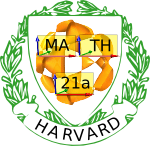6760, Math 21a, Fall 2009
Exhibits page Math 21a 2009, Multivariable Calculus
The super egg and other super surfaces
Office: SciCtr 434# The super egg

The super egg was designed by the Danish poet and scientist Piet Hein. in 1965. It is obtained by rotating a super ellipse around one of its axes.

What is the Super Egg? Lets first look at Super ellipses.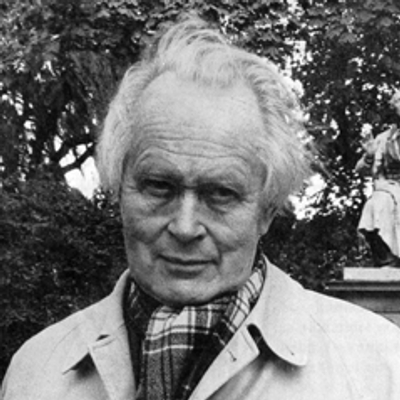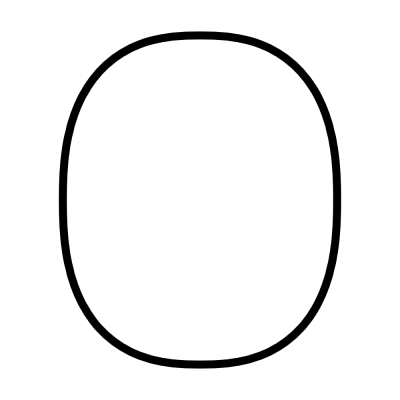## The Squircle and other Super ellipses

A super ellipse |x/a|e+|y/b|e = 1 around an x-axes. The number e is called the exponent of the super ellipse. For e=2, one obtains the usual ellipse. Super ellipses have been introduced by Gabriel Lamé. For exponents e smaller than 2, the super ellipse is called a hypoellipse, an example being e=2/3, the asteroid. For exponents e larger than 2, the super ellipse is called a hyperellipse an example being e=5/2, the Piet Hein ellipse. For a=b=1, we get "super circles". For e=4, in particular, one gets the squircle. Here is how one can plot a super ellipse with Mathematica:
```a=5;b=6;e=5/2; ContourPlot[Abs[x/a]^e+Abs[y/b]^e==1, {x,-7,7}, {y,-7,7}]
```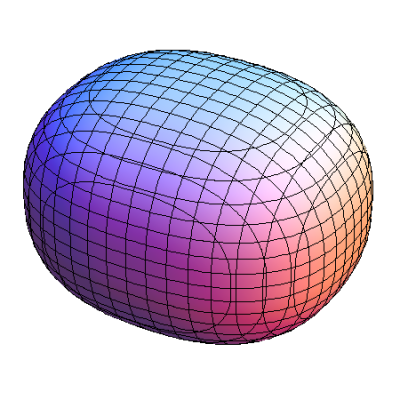## The Super egg and other Supers

A super ellipsoid is a surface with super ellipses as traces. It is defined implicitly as
```(|x|^e + |y|^e)^f/e + |z|^f = 1 .
```
It becomes the usual ellipsoid for e=f=2. You see that the x-trace as well as the y-traces are super ellipses with parameter f. The z-trace is a super ellipse with parameter e. Here is how we can draw a super ellipsoid with e=6/5 and f=5/2:
```e=6/5; f=5/2; ContourPlot3D[ (Abs[x]^e + Abs[y]^e)^(f/e) + Abs[z]^f == 1,  {x,-2,2},{y,-2,2},{z,-2,2}]
```
Of course, we can always deform and look more generally at surfaces of the type
```(|x/a|^e + |y/b|^e)^f/e + |z/c|^f = 1   .
```
These are the general super ellipsoids. For e=5/2,f=2, a/b = 6/5 and c=1 the super ellipsoid is called the super egg
```e=5/2; f=5/2; a=6/5; b=1; c=1;
ContourPlot3D[ (Abs[x/a]^e + Abs[y/b]^e)^(f/e) + Abs[z/c]^f == 1,  {x,-2,2},{y,-2,2},{z,-2,2}]
```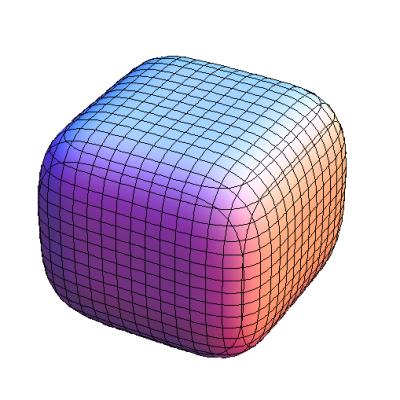Related to supper ellipsoids are super quadrics:
```|x/a|^e +|y/b|^f + |z/c|^g = 1
```
But the class of supper ellipsoids and super quadrics are different. The sets have intersections although Super ellipsoids with e=f are super quadrics with e=f. The super egg especially is a super quadric. Here is how one can draw super quadrics:
```e=6/5; f=5/2; g=3;
S = ContourPlot3D[ Abs[x]^e + Abs[y]^f + Abs[z]^g == 1,  {x,-2,2},{y,-2,2},{z,-2,2}]
```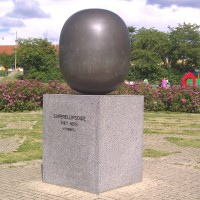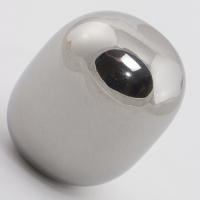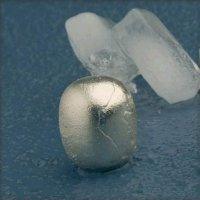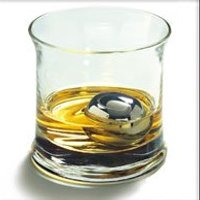## Is it useful?

It can be just beautiful. It can be seen as an art object. But the superegg is also sold as an ice cube for drinks. Why not just take a sphere? Well, ice cubes aren't cubes neither. And also, it would just not be so super any more. And sometimes, you just need a pickup line: "Do you know that the icecube in your drink is a very special surface? By the way, ..."? You get the idea.